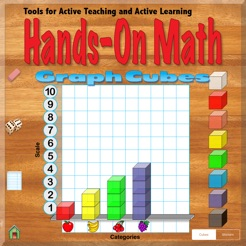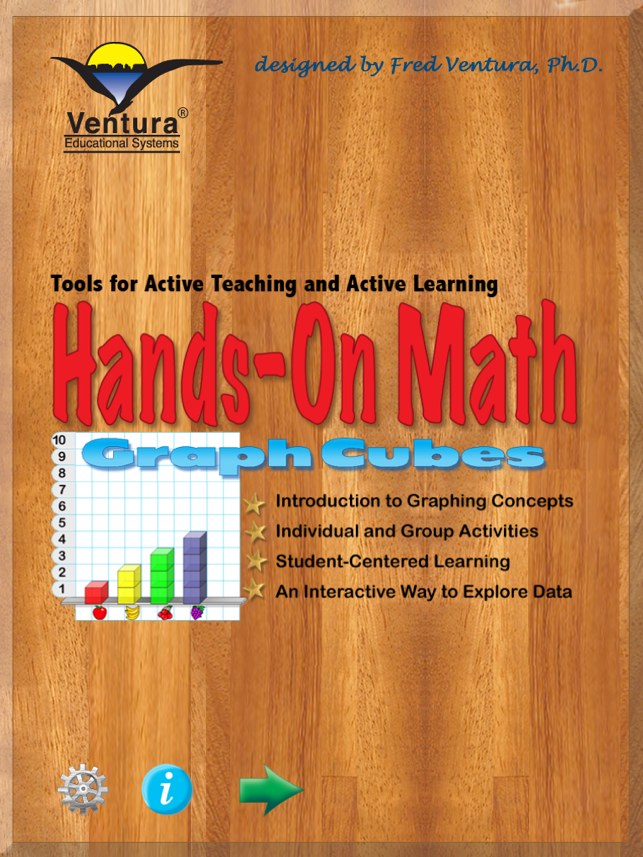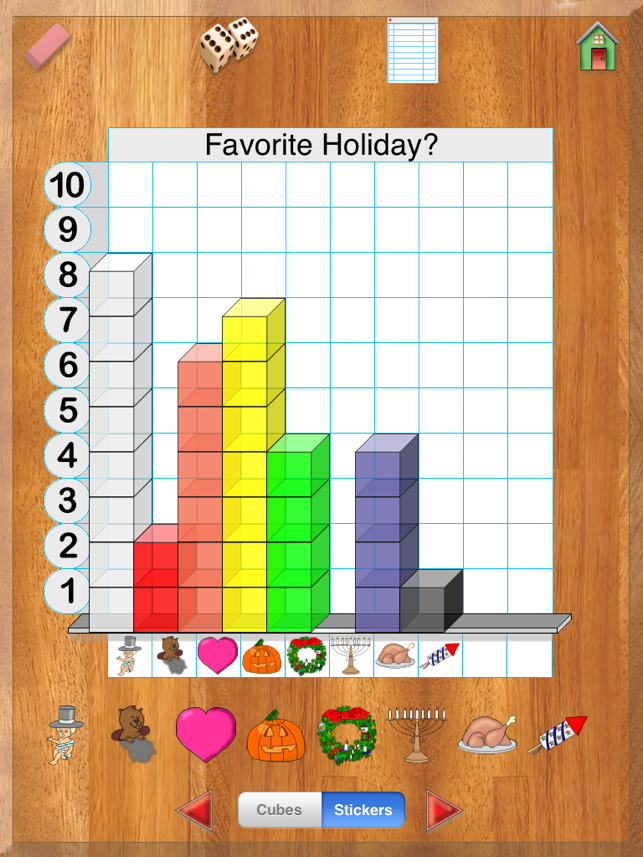## iPad Screenshots

•••••## Description

Hands-On Math Graph Cubes simulates the use of a set of cubes to build bar graphs. While using the cubes students are given feedback so that they can see logical relationships. Visualization is an effective way to help students understand mathematical reasoning and logic. A benefit derived from using the Graph Cubes is that the cubes can be easily manipulated and therefore good problem solving strategies spontaneously evolve. The concepts studied in this curriculum are the foundations of mathematical development especially in areas that are a part of the data analysis, probability and discrete mathematics curriculum strand. The app proivdes an interactive Playground where graphs and tables can be created and explored. Student interaction with the device is augmented by the artificially intelligent feedback provided by the software. The instructional approaches that result from creating an open-ended area for free exloration and discovery are exciting. On the Playground students manipulate a supply of virtual cubes to create graphs representing data collected through surveys.

When using Graph Cubes simple gestures are used to drag and drop blocks, placing them in columns to build bar graphs. The author and designer coined the term, “artificially intelligent math manipulative” to describe how using the Hands-On Math apps differs from the concrete manipulative devices traditionally used in classrooms. For example, this appprovides intelligent mathematical feedback as the student manipulates the cubes. Immediately associating feedback with a behavior is an effective way to reinforce behavior and facilitate learning.

Hands-On Math Graph Cubes focuses on these curriculum areas:

Collecting, Organizing, and Describing Data Based on Real-life Situations

Students may be expected to demonstrate such skills as:

• Analyze and describe data in terms of absolute or relative frequency of occurrence, range (highest/lowest), etc.
• Summarize information and relationships revealed by a graph.
• Add new data to a given chart, graph, or set of organized data.
• Extrapolate from obvious trends revealed by graphs in order to make predictions.

Using Graph Cubes students become involved in constructing, reading, and interpreting data in charts, graphs, tables, etc.

What students should know and be able to do includes:

• Obtain information from a bar graph by reading a graph to accurately to answer a question.
• Analyze, interpret, or draw conclusions based on data given in a bar graph.
• Match a graph to a described situation
• Match a data set to a graph and a graph to a data set (including matching data presented in a table/chart with a corresponding bar graph)
• Plot data onto a bar graph when the axes labels and scales have already been provided
• Draw a bar graph showing data given in a chart/, table or words

Formulating and solving problems that involve the use of data.

What students should know and be able to do includes:

• Generate questions that can be answered by collecting and analyzing data.
• Collect data to answer survey questions. (i.e., What is your favorite sport?)
• Solve problems using data from tables and graphs.
• Solve problems involving simple deductive reasoning. (e.g., "How many students chose 'dog' as their favorite pet?)

## What’s New

Version 2.3

This version provides support for the latest iOS versions.

5.0 out of 5
1 Rating

1 Rating

## Information

Seller
Ventura Educational Systems
Size
13.7 MB
Category
Education
Compatibility

Requires iOS 9.0 or later. Compatible with iPad.

Languages

English

Age Rating
Rated 4+
Copyright
©2018 Ventura Educational Systems - All Rights Reserved
Price
\$2.99

## Supports

•